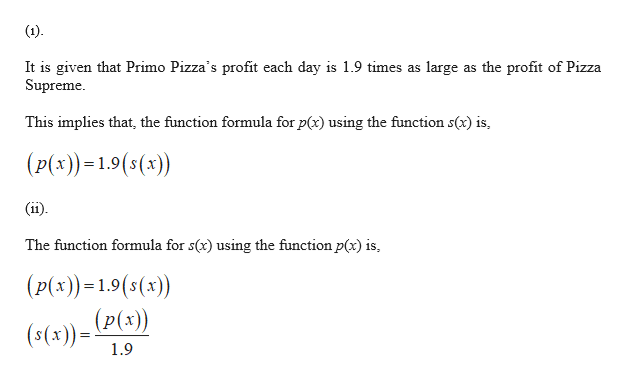# Each summer Primo Pizza and Pizza Supreme compete to see who has the larger summer profit. Let p(x) represent Primo Pizza's profit (in dollars) x days after June 1. Let s(x) represent Pizza Supreme's profit (in dollars) xdays after June 1.Suppose Primo Pizza's profit each day is 1.9 times as large as the profit of Pizza Supreme.Write a function formula for p using the function sp(x)= Write a function formula for s using the function p. Note that for this part the preview may look incorrect if you don't put an extra set of parentheses around your function notation, such as (f(x)) or (g(t)) s(x)= Suppose Primo Pizza's profit each day is \$220 more than the profit of Pizza Supreme.Write a function formula for p using the function s p(x)= Write a function formula for s using the function p s(x)= Suppose Primo Pizza's profit on a given day is always the same as the profit of Pizza Supreme's profit 4 days later.Write a function formula for p using the function s p(x)= Write a function formula for s using the function p s(x)=

Question
1007 views

Each summer Primo Pizza and Pizza Supreme compete to see who has the larger summer profit. Let p(x) represent Primo Pizza's profit (in dollars) x days after June 1. Let s(x) represent Pizza Supreme's profit (in dollars) x

days after June 1.

1. Suppose Primo Pizza's profit each day is 1.9 times as large as the profit of Pizza Supreme.

1. Write a function formula for p using the function s

p(x)=

• Write a function formula for s using the function p. Note that for this part the preview may look incorrect if you don't put an extra set of parentheses around your function notation, such as (f(x)) or (g(t))

s(x)=

Suppose Primo Pizza's profit each day is \$220 more than the profit of Pizza Supreme.

1. Write a function formula for p using the function s

p(x)=

• Write a function formula for s using the function p

s(x)=

Suppose Primo Pizza's profit on a given day is always the same as the profit of Pizza Supreme's profit 4 days later.

1. Write a function formula for p using the function s

p(x)=

• Write a function formula for s using the function p

s(x)=

check_circle

Step 1
1. Let p(x) represent Primo Pizza’s profit x days after June 1 and s(x) represent Pizza Supreme’s profit x days after June 1.help_outlineImage Transcriptionclose(1) It is given that Primo Pizza's profit each day is 1.9 times as large as the profit of Pizza Supreme This implies that, the function formula for p(x) using the function s(x) is (p(x))1.9(s(x)) (ii) The function formula for s(x) using the function p(x) is (p(x))=1.9(s(x)) (P(x)) (s(x) 1.9 fullscreen
Step 2
1.

(i). it is given that Primo Pizza’s profit each day ...

### Want to see the full answer?

See Solution

#### Want to see this answer and more?

Solutions are written by subject experts who are available 24/7. Questions are typically answered within 1 hour.*

See Solution
*Response times may vary by subject and question.
Tagged in

### Other